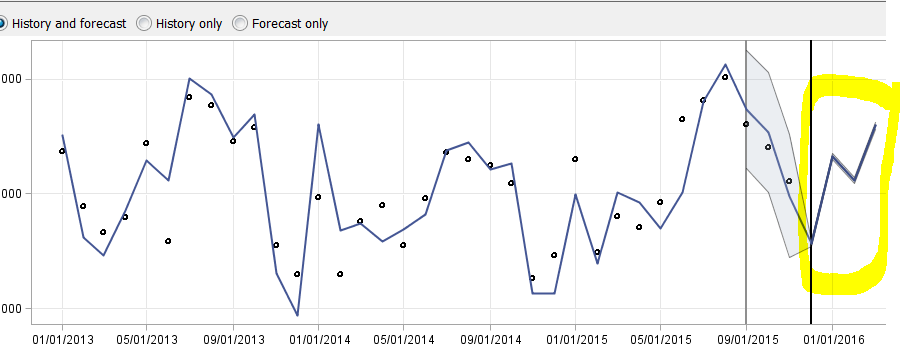## Getting confidence Intervals

I have monthly deactivation data  and i want to forecast 6 months into the future with SAS forecast studio for desktop. If  a month has more weekend days this year then deactivations will be lower / if less weekend days in the month the more deactivations. one way i thought of taking care of this is to calculate avg daily deactivations each month (based on working days of the month) then multiply the predictions by the working days in each predicted month. Question is How can i calculate the new Confidence Intervals?

Thanks

1 ACCEPTED SOLUTION

Accepted Solutions

## Re: Getting confidence Intervals

i just took a look that the data, i don't think the data in the forecast horizon periods are populated correctly.

1. I assumed the Region is the BY variable. There should be no missing values in the horizon periods.

2. You should populate the weekdays variable for each of the BY variable.

Please take a look at the attached worksheet with the correct horizon data.

11 REPLIES 11

## Re: Getting confidence Intervals

There are 2 approaches on top of my head:
1. create an input variable "number of weekend days" and incorporate it into the model (has to be either UCM or ARIMA)

2. Use the ADJUSTMENT option to first pre-adjust the monthly series, forecast the adjusted series, and post-adjust the forecasts. Forecast studio will compute the adjusted CI for you.

## Re: Getting confidence Intervals

I did that but have the following challenge: I have the number of working days for the months i have data how can SAS know the number of working days in the predicted months so the prediction is multiplied byt the number of working days?

## Re: Getting confidence Intervals

you will need to populate the input/adjustment variables for the forecast horizon periods, not just the historical periods.

## Re: Getting confidence Intervals

ok I did that seems to be working but i noticed the following with the C.I see attached. Very narrow,but not for the out of sample predictions, any explanation for that?## Re: Getting confidence Intervals

would you be able to share the data with me? Something is missing but it is hard to tell from the forecast plot. thanks

find attached.

thanks

## Re: Getting confidence Intervals

X1 is the dependent var

## Re: Getting confidence Intervals

i just took a look that the data, i don't think the data in the forecast horizon periods are populated correctly.

1. I assumed the Region is the BY variable. There should be no missing values in the horizon periods.

2. You should populate the weekdays variable for each of the BY variable.

Please take a look at the attached worksheet with the correct horizon data.

thank you!!!

## Re: Getting confidence Intervals

See if you can switch to more granular data at all, if you have daily data, it may be worth working at a week level.

## Re: Getting confidence Intervals

at this point can't get more granular data.

Discussion stats
• 11 replies
• 2026 views
• 0 likes
• 3 in conversation